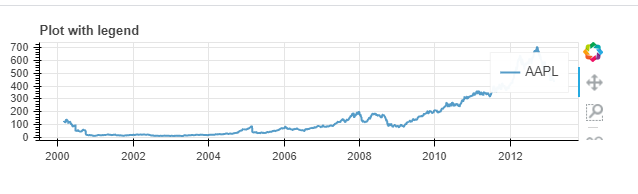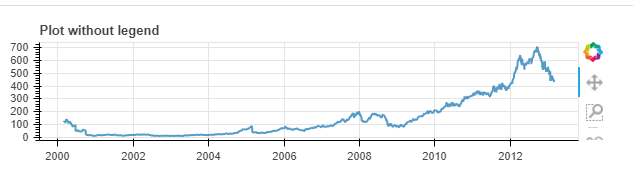GeeksforGeeks App
Open AppBrowser
Continue

Bokeh is a Python interactive data visualization. It renders its plots using HTML and JavaScript. It targets modern web browsers for presentation providing elegant, concise construction of novel graphics with high-performance interactivity. Adding legends to your figures can help to properly describe and define them. Hence, giving more clarity. Legends in Bokeh are simple to implement. They can be basic, automatically grouped, manually mentioned, explicitly indexed and also interactive. In this article we will discuss only how to have the legends completely invisible from the plot.

To achieve the required functionality we have to set the visible property of the bokeh legend to False.

Syntax:

bokeh.legend.visible=False

Approach

• Import module
• Create figure frame
• Plot required graph
• Set legend to invisible
• Display plot

First, let us see how a plot with legend looks like, and then we will set the visibility to false for the same plot.

Example

## Python3

 `# import module``import` `pandas as pd``from` `bokeh.plotting ``import` `figure, output_file, show``from` `bokeh.sampledata.stocks ``import` `AAPL`` ` `# create frame``pic ``=` `figure(plot_width``=``600``, plot_height``=``150``, x_axis_type``=``"datetime"``)``pic.title.text ``=` `'Plot without legend'`` ` `# plot data``for` `data, name, color ``in` `zip``([AAPL], [``"AAPL"``], Spectral4):``    ``df ``=` `pd.DataFrame(data)``    ``df[``'date'``] ``=` `pd.to_datetime(df[``'date'``])``    ``pic.line(df[``'date'``], df[``'close'``], line_width``=``2``,``             ``color``=``color, alpha``=``0.8``, legend_label``=``name)`` ` `# display plot``output_file(``"hide_legend.html"``, title``=``"hide_legend.py example"``)``show(pic)`

OutputExample: Without legend

## Python3

 `# import module``import` `pandas as pd``from` `bokeh.plotting ``import` `figure, output_file, show``from` `bokeh.sampledata.stocks ``import` `AAPL`` ` `# create frame``pic ``=` `figure(plot_width``=``600``, plot_height``=``150``, x_axis_type``=``"datetime"``)``pic.title.text ``=` `'Plot without legend'`` ` `# plot graph``for` `data, name, color ``in` `zip``([AAPL], [``"AAPL"``], Spectral4):``    ``df ``=` `pd.DataFrame(data)``    ``df[``'date'``] ``=` `pd.to_datetime(df[``'date'``])``    ``pic.line(df[``'date'``], df[``'close'``], line_width``=``2``,``             ``color``=``color, alpha``=``0.8``, legend_label``=``name)`` ` `# set visibility``pic.legend.visible ``=` `False`` ` `# print plot``output_file(``"hide_legend.html"``, title``=``"hide_legend.py example"``)``show(pic)`

OutputMy Personal Notes arrow_drop_up